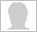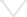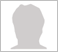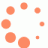0 out of 300 characters

QUESTION
1. They are to classes A&B;. In that classes some no. Of students.
From class A 10 student moved to B then the strength of A= strength of B.
20 students are moved from A to B then the strength of A=twice the strength of B
Above the both equations are independent then to find out how many students
By Ram prasad (Beginner)  5 years ago   14 views0 out of 1250 character

Report Abuse(Beginner) 5 years ago
40 students(Beginner) 5 years ago
(x-10)=(y+10)
2(x-20)=(y+20)
x-y=20
2x-y=60

2x-y=60
-
x-y=20
=>
x=40
n
y=20